International Journal of Science, Technology and Society
Volume 3, Issue 4, July 2015, Pages: 174-182

A Review of Distributed Generation Resource Types and Their Mathematical Models for Power Flow Analysis

Haruna Musa

Department of Electrical Engineering, Bayero University, Kano, Nigeria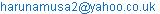(H. Musa)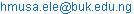(H. Musa)

Haruna Musa. A Review of Distributed Generation Resource Types and Their Mathematical Models for Power Flow Analysis. International Journal of Science, Technology and Society. Vol. 3, No. 4, 2015, pp. 174-182. doi: 10.11648/j.ijsts.20150304.21

Abstract: The emergence of Distributed Generation (DG) in distribution network has changed the configuration of this century’s power system in terms of power flow. The reason for this is that DG affects the power flow and voltage conditions in the distribution system; contrary to its traditional unidirectional nature in radial configuration. It is worth mentioning that the change in the direction of power flow is not limited to the distribution network, but can as well extend to the transmission or sub-transmission systems, especially when DG penetration is high. This paper gives an overview of DG types and modeling techniques of the DG for power flow analysis during planning and operations. Various DG technologies are highlighted, different models of DGs are presented and some key challenges ahead with current drive towards smart grid networks is also discussed.

Keywords: Distribution System, Modeling, Distributed Generation, Distributed Generation Resources, Power Flow, Power Converters

1. Introduction

The increasing demand for green energy sources to replace fossil fuel sources has brought some rapid increase in penetration of DG in power systems. The penetration is certainly going to increase to a level that would have an impact on the entire system operation and performance . DG penetration has increased more than 5 times during the last 8 years in some developing countries of Asia and this shows an increase that exceeds that of EU and US . Therefore, a study on cumulative effect of high penetration on the entire network at all levels is necessary. Even though DG is associated with benefits, there are a lot more technical issues that are still not well understood and addressed . For example, the studies conducted that relate interactions between transmission and distribution systems are based on simulations only. Unlike the simulations conducted on systems, real applications requires more adequate models of the DG units that can give better indication of the interactions in terms of power flows in the network. The models should meet certain basic requirements that can allow investigations at both local and global levels to be conducted. DG’s interaction has been studied from the local/micro-grid point of view to the overall/global system point of view level all with the aim of assessing its impacts on the overall network as the level of penetration increases. It was established that the impact of DG is no longer restricted to the local load or distribution network where these units are connected, but have impact on the entire transmission system . Alot of studies were conducted on large DG penetration that focuses on system control and stability.  However, these studies did not fully consider the various kinds of DG units, but mainly concentrated on stability aspect and control issues from the transmission system point of view. Thus, in  and , the impacts of DG penetration levels on power system transient stability are studied for different scenarios, and in , the impact of selected DG units, i.e. fuel cells (FCs) and micro turbines (MTs), on power system stability for various penetration levels were investigated. On the other hand, some studies have concentrated on the effect of DG units on the distribution network [7-10]. For example, in , the stability analysis of a distribution network with selected DG units, i.e. wind generators, and micro-turbines, was presented. Mathematical models of the DGs were integrated in three phase load flow algorithm and the special topological characteristics of distribution networks were fully utilized. The same algorithm was further improved to handle unbalanced three phase network based on ladder iterative method . The interesting aspect of the algorithm is its capability of handling more than one source without system constraints violation.

This paper presents an overview of DG types and models developed so far for the purpose of power flow analysis. Although there have been numerous publications on the topic, a specific review work on the methods and models has not taken place. The paper has highlighted the work done by researchers to date with contributions made and outlined the key challenges ahead that are yet to be addressed with current drive towards smart grid networks.

2. DG Resource Types

Generally Distributed generation resources are defined as those resources that are directly used in the generation of electric power for connection to distribution system. These sources include traditional and non-traditional such as renewable, non-renewable and energy storage technologies like batteries, flywheels, superconducting magnetic energy storage, to mention but a few as categorized in figure 1.0 with illustrations of various technologies.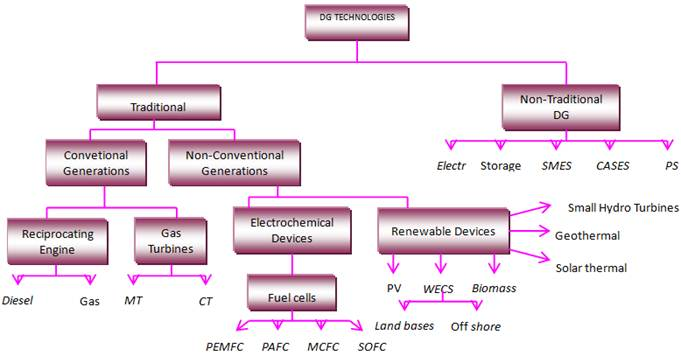Fig. 1. Distributed Generation Technologies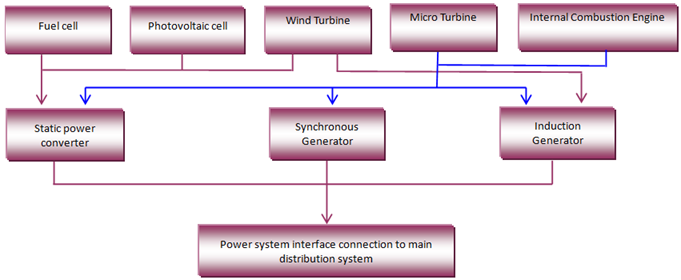Fig. 2. DGs and converter circuits connections for grid interfacing 

The traditional DGs are those generators that utilize combustion engines such as low speed turbines, reciprocating engine and gas micro-turbine. These resources even through are small in size but have wide spread geographically. On the other hand the Non-traditional DGs are those sources that produce power with zero emissions and are very friendly to environment. Most of these sources usually outputs DC power, therefore conversion to AC power is necessary before integration into an existing AC distribution network. For this reason sources such as photovoltaic and fuel cells uses power electronic converters (inverters) for grid interfacing as shown in figure 2.0.

The traditional internal combustion engines (rotary machines) are mainly synchronous generators and are interfaced directly to the grid. Wind turbines are also considered as rotary machines and are mainly induction type generators which can be interfaced directly with the grid. In some wind applications as well as some combustion engines like micro-turbines, power converter/inverter devices are employed for grid interface as the benefits associated with the electronic interface justifies the additional cost and complexity involved. Therefore, the energy generated from the various DG sources is injected into the grid via synchronous Generators, static power converters or induction generators.  The nature of operation of these generators or converters determines the models of DG to be employed in the power flow solution.

3. DG Resources and Conversion Devices Modeling

In general modelling requires system representation mathematically such that the mathematical model gives sufficient information about actual systems that covers all the necessary system behaviour within certain constraints. A proper model of the DG that can adequately represent a DG type with a view of assessing its impact on the network is of great importance. The model should be represented in such a way that the impact evaluation approach on the network due to the DG presence can easily be conducted. Many models have been developed by researchers on DG for load flow analysis in which the DGs are modeled as either a constant power factor model or constant voltage model or variable reactive power output power model . In their analysis the buses with DG connection that yields small output power are modeled as PQ nodes while those with large DG output are considered as PV nodes. Similar study was also conducted by  in which asynchronous and synchronous DG units that are connected to grid via power converters are modeled as either PV or PQ nodes depending on control technique employed. Other models were also discussed in  that are based on the control of generator excitation and in this case the synchronous DGs together with static voltage regulators are modeled as PV nodes.

Generally, DG classification can be based on its construction, size, and output power duration apart from classification based on technology earlier reported in . Also for the purpose of modelling DGs have been categorized into four classes be it traditional or non-traditional as suggested by authors in . The authors have classified the DGs into four major groups based on combined power transfer capability as well as terminal characteristics as presented;

1    Those DGs that can provide only active power (P) and can be integrated to the main grid by employing power converters are classified as one type. Typical examples of such are the fuel cell, photovoltaic, micro turbine and wind turbine.

2    The second classification of DG are those that are capable of providing both active (P) and reactive (Q) power. Such DG units type are based on synchronous machines which are commonly found in gas turbine and cogeneration.

3    The third are those that are only capable of supplying only reactive power. An example of such is the Synchronous compensators.

4    Some DGs can provide active power (P) and at the same time consumes reactive power (Q). The well known examples of such type are the induction generators and the doubly fed induction generator (DFIG) systems.

3.1. DG Models

Presence of DG in network means more active power supply to the network. Integration of DG sources such as photovoltaic, fuel cells, micro turbine and wind turbine systems into grid is via power electronic interfaces as shown in figure 2.1. For this reason modeling of DG units in load flow is always dependant on control technique that is been used in the converter circuit. The distribution system has some distinct characteristics which are always different from transmission systems in the sense that it is radial or weekly meshed with the lines having high R/X ratio and may be significantly unbalanced .

The sources of energy from the DGs can be categorized into stable energy sources (fuel cell, micro turbine and internal combustion engine) and unstable sources (wind and solar). The output characteristics of these sources are always dependant on the conversion unit employed. A typical example is when induction generator is used to convert wind energy in which case the output is a constant real power (P) with reactive power (Q). However, if static converter is used the output will be a constant power factor output in normal operation condition. Based on output characteristics of the sources, DG model can be classified as constant power factor model, constant voltage model or variable reactive power model as proposed by  and explained herein as follows;

A    Constant Power Factor Model

This type of model has a specified real and reactive power as well as power factor. The commonly used DGs that can be represented by such a model are the synchronous generators and power electronic based units that have outputs which can be adjusted by controlling the excitation current and trigger angles of the units for synchronous generator and power electronic converters respectively. The reactive power of such DGs can be calculated as presented in  based on;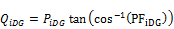(1)

while the injected DG equivalent currents is obtained as;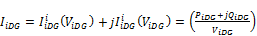(2)

where PiDG, and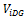are  the real output power and voltage at the terminal of the DG respectively, PFiDG is the power factor for the DG installed at bus i while QiDG is the calculated reactive power output.

B    Variable Reactive Power Model

Typical examples of such model are the induction generators that have variable reactive power generation. The real power output is dependent on the wind speed which is calculated based on wind turbine power curve, while the reactive power is dependent on real power output, and generator impedance. Even though the parameters are available, the calculation is usually cumbersome and difficult to execute efficiently. Hence the calculation is based on steady-state which can be represented as a function of the DG`s real power as in  by using;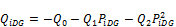(3)

whereis the reactive power function consumed by the wind turbine while the Q0, Q1 and Q2 are obtained experimentally. In situations when the reactive power required by the load cannot be supplied by the distribution network, capacitor banks are used to correct the power factor of the system. The reactive power output of the induction generator is expressed as;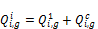(4)

where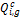is the reactive power supplied by capacitor bank.

C    Constant voltage model

The constant voltage model is meant for controllable DGs of large-scale systems where the specified parameters of this DG model are the real power output and bus voltage magnitude. The equivalent current to be injected is integrated into the power flow analysis after the reactive power output of the DG which is necessary to keep the bus voltage magnitude on the specified value are evaluated by using two-loop algorithm developed in . The generated output power after evaluating the required reactive power is given by;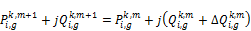(5)

where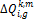is the required reactive power variation for the mth inner and kth outer iterations for the two-loops algorithm.

3.2. Power Flow with Distributed Generator

Many algorithms for power flow of distribution systems with high penetration of DG have been proposed by many researchers. Based on findings, it can be categorized into node based and branch based methods . In the node based methods, node voltage or current injections are employed as state variables for solving the power flow problems [24-26]. This method includes methods such as network equivalence method, Z-bus method, Newton-Raphson algorithm and fast decouple algorithm. On the other hand, the branch based involves use of branch currents or powers as the state variable for the power flow solution [27 – 29]. The technique is applicable to sweep based and loop impedance methods.

Since the DG units are modeled as either PQ or PV, their incorporation into power flow will involve use of active and reactive powers as flow variables instead of complex currents as variables. In the case of PV model the reactive power limits of the generator is constantly checked so that it is kept within limits otherwise the model will become a PQ model if demand for reactive goes beyond its limit. The root bus is considered as the slack bus with known voltage magnitude and angle. It most also be assumed that the initial voltage for all the other nodes are equal to the root node voltage and the initial power loss of all the branches be equal to zero. The integration of the mathematical models of DGs into the three-phase load flow can then be effectively conducted for the purpose of analysis based on the following models as in ;

3.2.1. Induction Generator Model

The Induction generator output power is always a function of two parameters which are slip and voltage and is expressed as;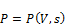(6)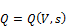(7)

where P and Q are the active and reactive powers produced respectively, s is the slip of the generator speed, and V is the bus voltage. By making an assumption that P is constant and neglecting the dependence of reactive power on the slip, equations (6) and (7) are now reduced to;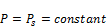(8)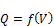(9)

The model of equations (8) and (9) are appropriate model for squirrel cage induction generator for power flow studies.

3.2.2. Synchronous Generator Model

Synchronous generator model is categorized into two types depending on the excitation system type. As shown Fig.3.0 the regulated excitation is modeled as either constant voltage (PV) or constant power factor model (PQ).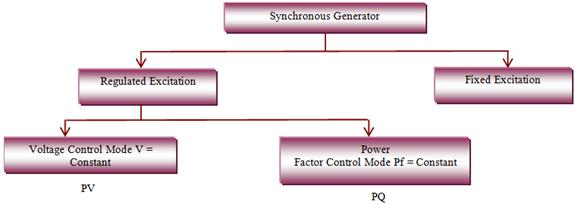Fig. 3. Synchronous generator model classification

The fixed excitation model can be described by equation (10);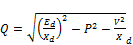(10)

where P and Q are the active and reactive power of DG respectively, Ed is the no-load voltage and is maintained constant, Xd is the synchronous reactance, V is the generator terminal voltage. Assuming that P is constant;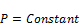(11)(12)

This model is the same as that in equation (8) and (9) except Q is positive in equation (12), which means the synchronous generator without excitation voltage regulation may inject reactive power to the grid.

Table 1. Methods, models and contributions on DG modelling.

3.2.3. Static Power Converter/Inverters Model

The static power converters are sometimes referred to as power electronic interface and are required for direct connection to grid. These power electronic devices are basically made of high power transistors and employs transistor switching techniques to control the flow of electric power. In this mode of operation at any instant of time, the transistor is either fully on or it is fully off. The transistors are configured into different topologies for the purpose of achieving the desired power conversion function such as DC-to-DC, AC-to-DC, or DC-to-AC. For such functions to be achieved pulse width modulation (PWM) is used for varying the on and off times of the transistors for a desired voltage and frequency. The modern transistors used for this purpose are made from insulated gate bipolar transistors (IGBT). As micro-sources like micro-turbines, wind turbines, photovoltaic array and even fuel cells  output powers are small DC (<100KW), power electronic interfaces are required for conversion  DC/AC or AC/DC/AC as shown in fig. 2.

The rule of thumb employed for the modeling of static power converters/inverters for power flow analysis by most researchers as proposed in [30,31] is the converter controls which will determine the type of model to be employed. If the converter is designed to control active power and Voltage independently then the model is PV and when it is designed to control active power and reactive power independently the model is a PQ node .

4. Summary of Current Works

In this section a summary of previous work done on DG modeling is presented together with methods adapted and models formulated as well as the contribution made from the studies conducted. The papers reviewed in Table 1.0 are not only limited to IEEE Xplore digital library but also to scienceDirect web site.

The reason for the summarized overview is to provide researchers at a glance the models already formulated and achievements made based on the methods already proposed. It can be observed that the models are formulated based on output characteristic of the DG, while the methods have touched on both balanced and unbalanced three phase  power systems. The unbalanced three phase system is a typical scenario of distribution networks.

5. Future Research and Challenges Ahead

The emerging smart grid concept that is likely going to affair in tomorrow’s distribution network requires repeated and fast load flow solutions for efficient planning, automation and optimization of power system. This therefore necessitates continuous development of appropriate models of DG sources involved in distribution networks power flow. Due to complex nature of some of the DG sources, researchers have employed the use of some assumptions in order to reduce the number of state variables for existing models developed. This situation suggests further work towards development of newer models that are based on state of art modeling techniques with more number of state variables for proper dynamic analysis of systems comes necessary. Hence, mathematical models are required that carries adequate information about the system for proper investigation of system performance depending on interest.

In general increase in penetration of DG requires formulation of new models of the power system that needs creation of decoupling power system analysis tool which can analyze the system using hybrid load flow.

Due to the unbalance nature of distribution networks, the concept of impedance matrix and nodal current injections used in generalized single equation load flow method cannot be employed as maintaining them constant during the analysis is impossible. This hinders the modeling of many components in distribution network. A new method that is more robust than commonly used forward/back sweep method is required which can allow inclusion of components such as transformers, voltage regulators, shunt capacitors and various types of loads.

It is evident that more challenges are likely to come up as network modeling has not been done with full details from network operator’s point of view. This means that it is only when these details are obtained and included in the models, that development of optimization and integration methods of DG resources can become an issue.

The techniques developed so far are only pointers towards finding answers to questions about the detailed characteristics of the existing models already developed along with the systematic approach to their allocation in distribution network during planning and operation. Newer modeling efforts are therefore required especially with the introduction of smart energy meters, electric vehicles and other demand side resources.

6. Conclusions

Review of the various DG modeling techniques for power flow analysis has been presented in this paper. Various types of DGs are considered for different model types depending on grid interfacing device employed. Also the power generated by the DG type to be injected into the grid and the characteristic of the interface device determines the type of DG model for power flow studies. Based on this, models are categorized into constant power factor model, constant voltage model and variable reactive power model. These models are developed for the purpose of integration into three- phase load flow analysis during planning and operations of distribution system.

On the whole the review has identified fruitful key areas of active research work for researcher’s reference and at the end the challenges ahead with current drive towards smart grid networks are outlined.

Acknowledgements

The author H. Musa acknowledges with gratitude the financial support in form of Research fellowship offered by Bayero University Kano Nigeria and the provision of suitable research facilities.

References

1. A. Narang, "Impact of large scale distributed generation penetration on power system stability," Natural Resources Canada, CETC, March 9, 2006.
2. K. K. Sharma, B. Singh "Distributed Generation- A New Approach" International Journal of Advanced Research in Computer Engineering & Technology (IJARCET) Volume 1, Issue 8, October 2012
3. T. Ackermann, G. Anderson, and L. Soder, ―Electricity market regulations and their impact on distributed generation,‖ in Proc. Conf,Electric Utility Deregulation and Restructuring and Power Technologies 2000, London, U.K., Apr. 4–7, 2000, pp. 608–613.
4. J.G. Slootweg, S. de Haan, H. Polinder, W. Kling, Modeling new generation and storage technologies in power system dynamics simulations, in: Proceedings IEEE Summer Meeting, Chicago, July 2002.
5. A. M. Azmy and I. Erlich, "Impact of distributed generation on the stability of electrical power system," in Proc. IEEE Power Engineering Society General Meeting, vol. 2, pp. 1056–1063, June 2005.
6. Syafii, K.M.Nor, M.Abdel-Akher, "Analysis of three phase distribution networks with distributed generation"IEEE 2nd International on Power and Energy Conference (PEC), pp.1563 – 1568, 2008.
7. Liu Qingzhen,Cai Jinding, "A Integrated Power Flow Algorithm for Radial Distribution System with DGs Based on Voltage Regulating" Asia-Pacific Power and Energy Engineering Conference (APPEEC) pp.1-4, 2010
8. M.Z.Kamh, R.Iravani,"A Unified Three-Phase Power-Flow Analysis Model For Electronically Coupled Distributed Energy Resources", IEEE Trans. on Power Delivery vol. 26 ,no. 2, pp. 899 – 909, 2011.
9. S.Elsaiah, M.Benidris,J.Mitra,"Power flow analysis of distribution systems with embedded induction generators"North American Power Symposium (NAPS),pp.1 – 6, 2012
10. S.Khushalani,N.Schulz, "Unbalanced Distribution Power Flow with Distributed Generation"IEEE PES Transmission and Distribution Conference and Exhibition, 2005/2006 PP. 301 – 306, 2006
11. Khushalani,J.M.Solanki,N.N.Schulz, "Development of Three-Phase Unbalanced Power Flow Using PV and PQ Models for Distributed Generation and Study of the Impact of DG Models" IEEE Trans on Power Systems, vol. 22 ,no. 3, pp. 1019 – 1025, 2007
12. Engineering guide for integration of distributed generation and storage into power distribution systems’, EPRI Technical Report TR-100419 Report, December 2000
13. Teng J.H., ―Modeling distributed generations in three phase distribution load flow,‖ IEE Proceeding Generation Transmission Distribution., vol. 2, no. 3, pp 330–340, 2008.
14. Moghaddas-Tafreshi S.M. and Mashhour E., ―Distributed generation modeling for power flow studies and a three-phase unbalanced power flow solution for radial distribution systems considering distributed generation,‖ Electrical Power Systems Research 79 (2009) pp 680–686.
15. Chen H., Chen J., Shi D. and Duan X., "Power flow study and voltage stability analysis for distribution systems with Distributed Generation" IEEE Power Engineering Society Meeting 10-22 June, 2006, pp 8.
16. El-Khattam W., and Salama M. M. A, "Distributed Generation Technologies, Definitions and Benefits" Electric Power System Research Vol. 71, no. 2, pp. 119-128 2004
17. Hung D. Q., Mithulananthan N., and Bansal R. C., "Analytical expression for DG allocation in primary distribution network," IEEE Trans. Energy Convers., vol. 25, no. 3, pp. 814-820, 2010.
18. Y. Zhu, K. Tomsovic, Adaptive power flow method for distribution systems with dispersed generation, IEEE Trans. Power Deliv. 17 (3) (2002) 822–827.
19. J.-H. Teng, "Modelling distributed generations in three-phase distribution load flow" IET Gener. Transm. DistribVol. 2, No. 3, pp. 330–340, 2008
20. Chen Th, Chen Ms, Inoue T. ‘Three-phase cogenerator and transformer models for distribution system analysis’, IEEE Trans. Power Deliv., 1991, 6, (4), pp. 1671–1681
21. Feijoo Ae, Cidras J ‘Modeling of wind farms in the load flow analysis’, IEEE Trans. Power Syst., 2000, 15, (1), pp. 110–115
22. Teng Jh: ‘A direct approach for distribution system load flow solutions’, IEEE Trans. Power Deliv., 2003, 18, (3), pp. 882–887
23. W.C. Wu, B.M. Zhang, A three-phase power flow algorithm for distribution system power flow based on loop-analysis method, Elect. Power Energy Syst. 30 (2008) 8–15.
24. A.V. Garcia, M.G. Zago, Three phase fast decoupled load flow for distribution networks, IEEE Proc – Gener. Transm. Distrib. 143 (2) (1996) 188–192.
25. P.A.N. Garcia, J.L.R. Pereira, "Three-phase power flow calculations using the current injection method", IEEE Trans. Power Syst. 15 (2) (2000) 508–514.
26. J.-H. Teng, C.-Y. Chang, A novel and fast three-phase load flow for unbalanced radial distribution systems, IEEE Trans. Power Syst. 17 (4) (2002) 1238–1244.
27. A.G. Bhutad, S.V. Kulkarni, S.A. Khaparde, Three-phase load flow methods for radial distribution networks, in: Conf. on Convergent Technologies for Asia- Pacific Region, TENCON 2, 2003, pp. 781–785.
28. D. Thukaram, H.M.W. Banda, I. Jerome, "A robust three-phase power flow algorithm for radial distribution systems", Elect. Power Syst. Res. 55 (3) (2000) 191–200.
29. R. Ranjan, B. Venkatesh, A. Chaturvedi, D. Das, "Power flow solution of three phase unbalanced radial distribution networks", Elect. Power Comp. Syst. 32 (4) (2004) 421–433.
30. S. Naka, T. Genji, Y. Fukuyama, "Practical equipment models for fast distribution power flow considering interconnection for distributed generators", IEEE Power Engineering Society Summer Meeting, vol. 2, 2001, pp. 1007–1012.
31. Pecas Lopes J.A., Moreira C.L, Madureira A.G., " Defining control strategies for MicroGrids islanded operation" IEEE Trans Power System, vol. 21no.2, pp.916–24, 2006.
32. H. Chen, J. Chen, D. Shi, X. Duan, "Power flow study and voltage stability analysis for distribution systems with distributed generation", IEEE Power Engineering Society General Meeting, pp.8-12, 18–22 June, 2006
33. G.P.Harrison,A.R.Wallace, "Optimal power flow evaluation of distribution network capacity for the connection of distributed generation" IEE Proceedings-Generation, Transmission and Distributionvol. 152 ,no. 1 pp. 115 – 122, 2005
34. D.N. Hussein, M. El-Syed, H.A. Attia, "Modeling and simulation of distributed generation (DG) for distribution systems load flow analysis"Eleventh International Middle East Power Systems Conference (MEPCON 2006)vol. 1 pp.285 – 291, 2006
35. D.Gayme,Ufuk Topcu, "Optimal power flow with distributed energy storage dynamics"American Control Conference (ACC),pp.1536 – 1542, 2011
36. M.Z. Kamh, R. Iravani, "Three-Phase Steady-State Model of Type-3 Wind Generation Unit—Part I: Mathematical Models"IEEE Trans. Sustainable Energy, vol. 2, no. 4, pp. 477-486, 2011
37. Hany E. Farag, E.F. El-Saadany, Ramadan El Shatshat, Aboelsood Zidan,"A generalized power flow analysis for distribution systems with high penetration of distributed generation" Electric Power Systems Research vol. 81, pp.1499–1506, 2011
38. M.Z.Kamh, R.Iravani,"Three-Phase Steady-State Model of Type-3 Wind Generation Unit—Part II: Model Validation and Applications" IEEE Trans. Sustainable Energy, vol. 3, no. 1, pp. 41-48, 2012.
39. S.K.Nayak, D.N.Gaonkar,"Modeling and performance analysis of micro-turbine generation system in grid connected/islanding mode" IEEE International Conference on Power Electronics, Drives and Energy Systems (PEDES), Page(s): 1 – 6, 2012
40. J.M.Sexauer,; S.Mohagheghi,"Voltage Quality Assessment in a Distribution System With Distributed Generation—A Probabilistic Load Flow Approach" IEEE Trans.Power Deliveryvol. 28 ,no. 3 pp.1652 – 1662, 2013
41. Antonios G. Marinopoulos, Minas C. Alexiadis, Petros S. DokopouloN "Energy Losses in a distribution line with distributed generation based on stochastic power flow" Electric Power Systems Research vol. 81, pp. 1986– 1994, 2011
42. F.J. Ruiz-Rodriguez, J.C. Hernández, F. Jurado, "Probabilistic load flow for photovoltaic distributed generation using the Cornish–Fisher expansion" Electric Power Systems Research vol. 89, pp.129– 138, 2012
43. M. F. Akorede, H. Hizam, and E. Pouresmaeil, "Distributed energy resources and benefits to the environment," Renewable and Sustainable Energy Reviews, vol.14, pp. 724-734, 2010.

 Contents 1. 2. 3. 3.1. 3.2. 4. 5. 6.
Article ToolsAbstractPDF(376K)